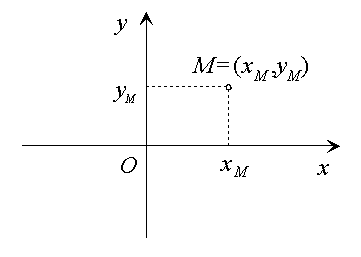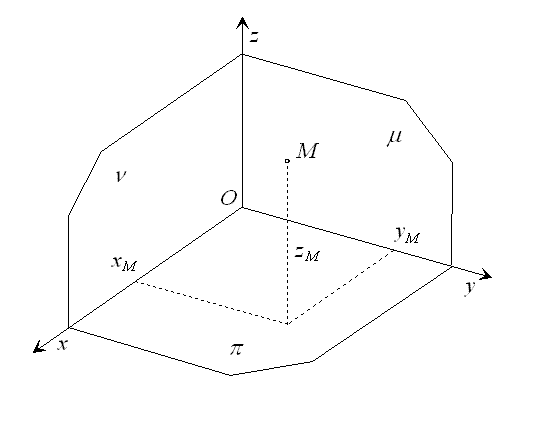# Cartesian coordinate system

Definition 1. Non-empty set ${\mathbf{E}}^{n}\left(R\right)$ is called the n-dimensional Euclidean space defined over the set of real numbers iff

1. any two points X,Y from the space can be attached a unique real number $|XY|$ - their distance

2. there exists at least one one-to-one mapping of this set to the set of all ordered n-tuples of real numbers such, that if the point X is attached n-tuple $\left({x}_{1},...,{x}_{n}\right)$ and point Y n-tuple $\left({y}_{1},...,{y}_{n}\right)$, then

 $|XY|=\underset{i=1}{\overset{n}{\sum \sqrt{{\left({x}_{i}-{y}_{i}\right)}^{2}}}}$

Distance of points $|XY|$ in the Euclidean space satisfies some important properties.

1. For any two points in the space ${\mathbf{E}}^{n}\left(R\right)$ the relation $|XY|\geqq 0$ is true and $|XY|=0,\phantom{\rule{2mm}{2mm}}\mathrm{if}\phantom{\rule{2mm}{2mm}}X=Y$.
2. For any two points in the space ${\mathbf{E}}^{n}\left(R\right)$ the equality $|XY|=|YX|$ holds.
3. For any three points in the space ${\mathbf{E}}^{n}\left(R\right)$ the triangular inequality $|XY|+|YZ|\geqq |XZ|$ holds.

The one-to-one mapping as defined in the Definition 1. is called the Cartesian coordinate system in the n-dimensional Euclidean space ${\mathbf{E}}^{n}\left(R\right)$. Origin of the coordinate system is the point attached the n-tuple consisting from zeros only.

Line is one-dimensional Euclidean space ${\mathbf{E}}^{1}\left(R\right)$, plane is two-dimensional Euclidean space ${\mathbf{E}}^{2}\left(R\right)$ and ${\mathbf{E}}^{3}\left(R\right)\phantom{\rule{2mm}{2mm}}$is three-dimensional Euclidean space.

In the Euclidean plane ${\mathbf{E}}^{2}\left(R\right)$, the Cartesian coordinate system $\left(O,\phantom{\rule{2mm}{2mm}}x,\phantom{\rule{2mm}{2mm}}y\right)$ can be defined, while:

1. O be an arbitrary fixed point called the origin of the coordinate system,
2. x and y called coordinate axes be two perpendicular lines sharing the common point O.Figure 1: Cartesian coordinates of point in plane

Choosing the positive orientation on the half-lines with the start point O and the measurement unit for the length on the coordinate axes x and y, any point M in the plane can be attached a unique ordered pair of real numbers $M=\left({x}_{M},\phantom{\rule{2mm}{2mm}}{y}_{M}\right)$ called Cartesian coordinates of the point in plane.

The two coordinates ${x}_{M},\phantom{\rule{2mm}{2mm}}{y}_{M}$ determine distances of he point M from the coordinate axes y and x respectively.

In the Euclidean space ${\mathbf{E}}^{3}\left(R\right)$, the Cartesian coordinate system $\left(O,\phantom{\rule{2mm}{2mm}}x,\phantom{\rule{2mm}{2mm}}y,\phantom{\rule{2mm}{2mm}}z\right)$ can be defined, while:

1. O be an arbitrary fixed point called the origin of the coordinate system,
2. x, y and z called coordinate axes be three perpendicular lines sharing the common point O, and forming 3 coordinate planes $\pi =xy,\phantom{\rule{2mm}{2mm}}\nu =xz,\phantom{\rule{2mm}{2mm}}\mu =yz$.Figure 2: Cartesian coordinate system in space

Choosing the positive orientation on the half-lines with the start point O and the measurement unit for the length on the coordinate axes x, y and z, any point M in the space can be attached a unique ordered triple of real numbers

 $M=\left({x}_{M},{y}_{M},{y}_{M}\right)$

called Cartesian coordinates of the point in space. The three coordinates ${x}_{M},\phantom{\rule{2mm}{2mm}}{y}_{M},\phantom{\rule{2mm}{2mm}}{z}_{M}$ determine distances of he point M from the coordinate planes $\mu ,\phantom{\rule{2mm}{2mm}}\nu \phantom{\rule{2mm}{2mm}}\mathrm{and}\phantom{\rule{2mm}{2mm}}\pi$ respectively.